## Saturday, 19 November 2011

### NSS Mathematics: Co-geom techniques

﻿
This is a question from the latest test. A, B H are points (-6,0), (4,0), (0,10) respectively. C1 and C2 are circles with AO and BO as diameter respectively, AH and BH meet C1 and C2 at F and G respectively.
ai) Show OGHF concyclic.
ii) Show AFGB concyclic.
bi) Find coordinate of G.
ii) Find circle containing AFGB.

We will focus on part b as it includes all method that we can solve a problem related to circle in the co-geom plane.

bi) Find coordinate of G.

Method 1: Circle-line intersection
Recall the equation of C2: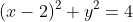Equation of line BH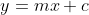, putting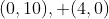we get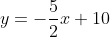.
Putting equation of line BH into equation C2: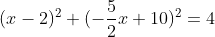.
By simplification we have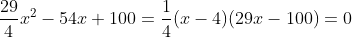. (4,0) is B so x=4 is rejected. Then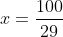, and we can easily get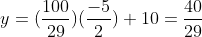.

Method 2: (the easiest one) perpendicular line interesection
Observe that OG is perpendicular to BH due to angles in semi-circle,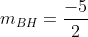,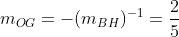. Since OG passes through the origin,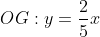.
The intersection between line BH and line OG: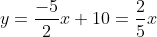, we can easily obtain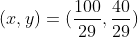.

Method 3: perpendicular line-circle intersection
The intersection between OG and C2 might me a bit easier than intersection between BH and C2: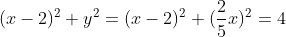, x = 0 (rej. since it's O) and the same result as before.

Method 4: trigonmetry method
Observe the triangles HOB, HGO and OBG are similar. Let angle GOB = angle HOB be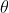and the coordinate of G is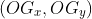.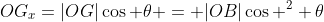, similarly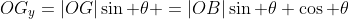.
By definition we have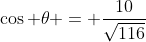,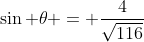, by putting |OB| = 4 we have the same result.

(Note: |XY| is the length of line segment XY.)

bii) Find the equation of circle:

Method 1: general equation of circle
Assume the equation is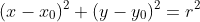.
Putting point A and B we have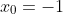, therefore the equation becomes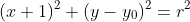and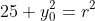.
Putting, we have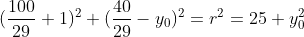After a bunch of complex calculation (ugly number), we have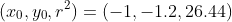, therefore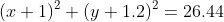is the desired equation.

Method 2: Perpendicular bisector method
Recall the way you determine the circumcenter: it's the intersection point among three perpendicular bisectors. Therefore if four points are concyclic, choose two perpendicular bisector of them, then insection point is the center of circle. The radius can be easily determined by distance formula.

The perpendicular bisector of AB is trivially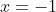.
The perpendicular bisector of BG is given by the locus of P that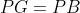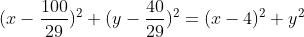By putting x = -1, we have y = -6/5, and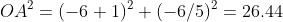, and the same result is given.

Conclusion:
1) Change circle problems into linear problems whenever possible.
2) Trigonometry is powerful when there's perpendicular pair of lines.
3) Finding intersection between circles is stupid.
4) Find the equation of circle in terms of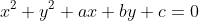is stupid.
5) Perpendicular bisector is our new method to find equation of circle.

fin.